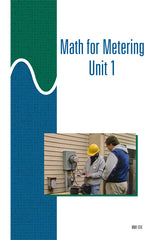#### Get the Study Guide for this videoFollow the video session-by-session with this handy book. Color illustrations throughout. \$15

Instructor Guide  available

# Math for Metering 1 - DVD

### \$ 145.00

##### Summary

This 30-minute DVD introduces the concepts of ratios, percent's, squares, and square roots. Also includes demonstrations of how to manipulate simple algebraic equations.

##### Training Sessions

Roots and Powers – 8 minutes
Defines: root, square root, power, exponent. Describes what powers are and how they are indicated. Explains how to add, subtract, multiply, and divide numbers taken to various powers. Explains how powers of 10 are related to place values.

Number Relationships – 8 minutes
Defines: ratio, direct proportion, inverse proportion, percentage, average. Describes how ratios can be expressed in mathematical terms. Explains how to convert between percent's, fractions, and decimal numbers. Explains how averages are calculated.

Equations – 8 minutes
Explains the basic difference between arithmetic operations and algebraic operations. Describes two rules for solving equations. Shows how the components of an equation can be rearranged to solve for a desired value. Explains the terms “combining like terms” and “distribution.”

Metering Examples – 6 minutes
Describes several ways in which math can be used in day-to-day metering applications.

Item Code: MM1-DVD